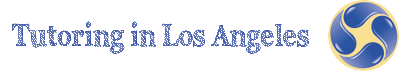# Algebra I and II Tutoring

Tutoring in Los Angeles helps students to use symbolic reasoning and calculation through the study of Algebra. Students with the help of our tutors will be able to develop algebraic skills and use them in problem-solving situations.

Symbolic reasoning and calculations with symbols are central in algebra. Through the study of algebra, a student develops an understanding of the symbolic language of math and the sciences. In addition, algebraic skills and concepts are developed and used in a wide variety of problem-solving situations. The key content for the first course, Algebra I, involves understanding, writing, solving, and graphing linear and quadratic equations, including systems of two linear equations in two unknowns.

Algebra proper again starts informally. It appears initially in its proper form in the third grade as "generalized arithmetic." In later grades algebra is the vital tool needed for solving equations and inequalities and using them as math models of real situations. Students solve the problems that arise by translating from natural language-by which they communicate daily-to the abstract language of algebra and, conversely, from the formal language of algebra to natural language to demonstrate clear understanding of the concepts involved.

Algebra I is a gateway course. Without a strong background in the fundamentals of algebra, students will not succeed in more advanced mathematics courses such as calculus. An algebra readiness course will prepare students for success in algebra and subsequent advanced courses.

Algebra 2 complements and expands the math content and concepts of algebra I and geometry. Students who master algebra II will gain experience with algebraic solutions of problems in various content areas, including the solution of systems of quadratic equations, logarithmic and exponential functions, the binomial theorem, and the complex number system.

The general goal in Linear Algebra is for students to learn the techniques of matrix manipulation so that they can solve systems of linear equations in any number of variables. Linear algebra is most often combined with another subject, such as trigonometry, math analysis, or precalculus.

Standards are provided for Algebra I, geometry, Algebra II, trigonometry, math analysis, linear algebra, probability and statistics, AP probability and statistics, and calculus. Many of the more advanced subjects are not taught in every middle school or high school. Moreover, schools and districts have different ways of combining the subject matter in these various disciplines.

For example, many schools combine some trigonometry, mathematical analysis, and linear algebra to form a precalculus course. Some districts prefer offering trigonometry content with Algebra II.

 Phone: (866) 414 2030 Toll Free (310) 405 0721 (818) 850 2030 Fax: (866) 669 9584 Email: tutor@tutoringinla.com